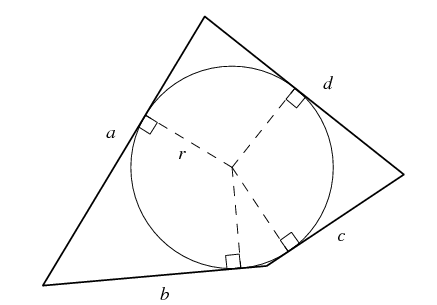In geometry, the tangential quadrilateral is a convex quadrilateral whose sides are all tangent to a single circle within the quadrilateral. This circle is called the incircle of the quadrilateral or its inscribed circle, its center is the incenter and its radius is called the inradius.

Since these quadrilaterals can be drawn surrounding or circumscribing their incircles, they have also been called circumscribable quadrilaterals, circumscribing quadrilaterals, and circumscriptible quadrilaterals. Tangential quadrilaterals are a special case of tangential polygons.Let a convex quadrilateral with sides a, b, c, d, then the area of a Tangential quadrilateral is, a + c = b + d

$\large area=\sqrt{abcd}$

Or the formula can also be written as

$\large A=rs$

Where,
r = radius of inscribed circle
s = semi-perimeter = (a + b + c + d)

### Examples of Tangential Quadrilateral Formula

Example: Find the measure of the fourth side of a quadrilateral circumscribed about a circle,  if three other sides have the measures of  5 cm,  6 cm  and  4 cm  listed consecutively.

Solution

Let  x  be the measure of the fourth side of our quadrilateral. Since the quadrilateral is circumscribed about a circle, the sums of the measures of its opposite sides are equal. Thus, you can write the equation:

5 + 4 = 6 + x
From this equation,  x = 5 + 4 – 6 = 3

Answer: The fourth side of the quadrilateral is of  3 cm  long.